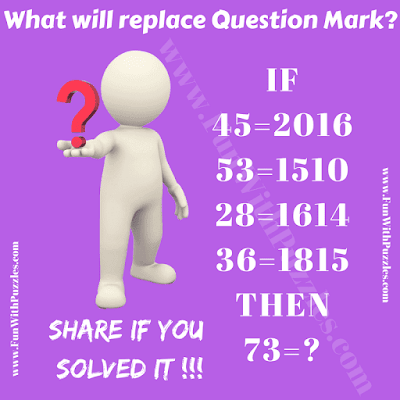Calling all school-going teens for a captivating math challenge that will put your problem-solving skills to the test! Our Teen Math Mind Quiz Puzzle is designed to engage young minds in a stimulating puzzle that combines math and logical reasoning.Teen Math Mind Quiz Puzzle: Decode the Number Equations

Within this intriguing puzzle, you'll encounter a series of number equations that may initially appear perplexing. The statement reads: "If 45=2016, 53=1510, 28=1614, 36=1815, then 73=?". Each of these number equations follows a unique mathematical relationship between the numbers on either side of the equation.

Your mission is to decipher this hidden mathematical relationship and calculate the missing value represented by the question mark. This challenge not only tests your math skills but also encourages critical thinking and pattern recognition.

Solving this puzzle requires more than just math; it's about unraveling complex patterns and understanding the logic behind the equations. As you engage with this challenge, you'll enhance your problem-solving abilities and strengthen your mathematical skills.

So, how quickly can you crack the code and solve the puzzle? Dive into this mind-bending math challenge, put your math prowess to the test, and celebrate your success as you uncover the hidden mathematical patterns.

The answer to this "Math Mind Quiz Puzzle for Teens", can be viewed by clicking on the button. Please do give your best try before looking at the answer.

Unknown said...

(4*5)((4*5)-4)=2016
(5*3)((5*3)-5)=1510
(2*8)((2*8)-2)=1614
(3*6)((3*6)-3)=1815
(7*3)((7*3)-7)=2114

Unknown said...

VERY SIMPLE but took me 2 minutes atleast to figure out

Anonymous said...

3630

Unknown said...

7×3=21
21-3=18
=2118 Ans💯